SYLLABUS  Previous: 1.3 Prototype problems  Up: 1.3 Prototype problems  Next: 1.3.2 Diffusion

Also called convection, advection models the streaming of infinitesimal elements in a fluid. It generally appears when a transport process is modeled in a Eulerian representation using the convective derivative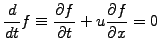(1.3.1#eq.1)

For a constant advection velocity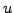, the advection equation can be solved analytically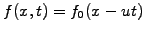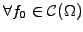, showing explicitly the underlying characteristic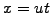. Try the JBONE applet below to compute the advection of a Gaussian pulse using the Lagrangian CIP method from chapter 6.

 Numerical experiments: advection Switch the initial condition from a Gaussian to Cosine and Box functions. Observe how the sharp edges from the box disappear in the numerical solution; this is in clear contradiction with the analytical solution. Reduce the Velocity input parameter and verify that the advection speed is reduced accordingly. Increase the velocity above unity and determine the threshold above which the numerical solution clearly becomes unstable.

Both experiments show that numerical simulations have to be used carefully, to work withing limits of applicability that will be discussed in the comming sections. Note that for a constant advection speed, the wave equation can be written in flux-conservative form that reminds an advection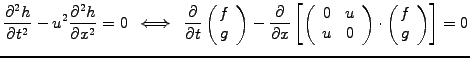(1.3.1#eq.2)

This shows explicitly that the numerical methods for the advection equation can in principle be used also for wave problems.

SYLLABUS  Previous: 1.3 Prototype problems  Up: 1.3 Prototype problems  Next: 1.3.2 Diffusion